Next: Classical Linear Transformations Up: Survey on Independent Component Previous: Survey on Independent Component

# Introduction

A central problem in neural network research, as well as in statistics and signal processing, is finding a suitable representation of the data, by means of a suitable transformation. It is important for subsequent analysis of the data, whether it be pattern recognition, data compression, de-noising, visualization or anything else, that the data is represented in a manner that facilitates the analysis. As a trivial example, consider speech recognition by a human being. The task is clearly simpler if the speech is represented as audible sound, and not as a sequence of numbers on a paper.

In this paper, we shall concentrate on the problem of representing continuous-valued multidimensional variables. Let us denote by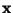an m-dimensional random variable; the problem is then to find a function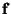so that the n-dimensional transform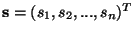defined by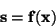(1)

has some desirable properties. (Note that we shall use in this paper the same notation for the random variables and their realizations: the context should make the distinction clear.) In most cases, the representation is sought as a linear transform of the observed variables, i.e.,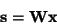(2)

where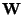is a matrix to be determined. Using linear transformations makes the problem computationally and conceptually simpler, and facilitates the interpretation of the results. Thus we treat only linear transformations in this paper. Most of the methods described in this paper can be extended for the non-linear case. Such extensions are, however, outside the scope of this paper.

Several principles and methods have been developed to find a suitable linear transformation. These include principal component analysis, factor analysis, projection pursuit, independent component analysis, and many more. Usually, these methods define a principle that tells which transform is optimal. The optimality may be defined in the sense of optimal dimension reduction, statistical 'interestingness' of the resulting components si, simplicity of the transformation, or other criteria, including application-oriented ones.

Recently, a particular method for finding a linear transformation, called independent component analysis (ICA), has gained wide-spread attention. As the name implies, the basic goal is to find a transformation in which the components si are statistically as independent from each other as possible. ICA can be applied, for example, for blind source separation, in which the observed values ofcorrespond to a realization of an m-dimensional discrete-time signal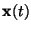, t=1,2,.... Then the components si(t) are called source signals, which are usually original, uncorrupted signals or noise sources. Often such sources are statistically independent from each other, and thus the signals can be recovered from linear mixtures xi by finding a transformation in which the transformed signals are as independent as possible, as in ICA. Another promising application is feature extraction, in which si is the coefficient of the i-th feature in the observed data vector. The use of ICA for feature extraction is motivated by results in neurosciences that suggest that the similar principle of redundancy reduction explains some aspects of the early processing of sensory data by the brain. ICA has also applications in exploratory data analysis in the same way as the closely related method of projection pursuit.

In this paper, we review the theory and methods for ICA. First, we discuss relevant classical representation methods in Section 2. In Section 3, we define ICA, and show its connections to the classical methods as well as some of its applications. In Section 4, different contrast (objective) functions for ICA are reviewed. Next, corresponding algorithms are given in Section 5. The noisy version of ICA is treated in Section 6. Section 7 concludes the paper. For other reviews on ICA, see e.g. [3,24,95].Next: Classical Linear Transformations Up: Survey on Independent Component Previous: Survey on Independent Component
Aapo Hyvarinen
1999-04-23